# Data analysis using Pandas

• Difficulty Level : Medium
• Last Updated : 15 Oct, 2020

Pandas is the most popular python library that is used for data analysis. It provides highly optimized performance with back-end source code is purely written in C or Python.

```We can analyze data in pandas with:

SeriesDataFrames```

### Series:

Series is one dimensional(1-D) array defined in pandas that can be used to store any data type.

Attention geek! Strengthen your foundations with the Python Programming Foundation Course and learn the basics.

To begin with, your interview preparations Enhance your Data Structures concepts with the Python DS Course. And to begin with your Machine Learning Journey, join the Machine Learning - Basic Level Course

Code #1: Creating Series

 `# Program to create series`` ` `# Import Panda Library``import` `pandas as pd  `` ` `# Create series with Data, and Index``a ``=` `pd.Series(Data, index ``=` `Index)  `

Here, Data can be:

1. A Scalar value which can be integerValue, string
2. A Python Dictionary which can be Key, Value pair
3. A Ndarray

Note: Index by default is from 0, 1, 2, …(n-1) where n is length of data.

Code #2: When Data contains scalar values

 `# Program to Create series with scalar values `` ` `# Numeric data``Data ``=``[``1``, ``3``, ``4``, ``5``, ``6``, ``2``, ``9``]  `` ` `# Creating series with default index values``s ``=` `pd.Series(Data)    `` ` `# predefined index values``Index ``=``[``'a'``, ``'b'``, ``'c'``, ``'d'``, ``'e'``, ``'f'``, ``'g'``] `` ` `# Creating series with predefined index values``si ``=` `pd.Series(Data, Index) `

Output:

```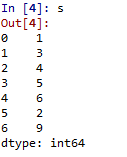Scalar Data with default Index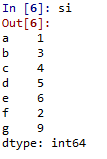Scalar Data with Index
```

Code #3: When Data contains Dictionary

 `# Program to Create Dictionary series``dictionary ``=``{``'a'``:``1``, ``'b'``:``2``, ``'c'``:``3``, ``'d'``:``4``, ``'e'``:``5``} `` ` `# Creating series of Dictionary type``sd ``=` `pd.Series(dictionary) `

Output:

```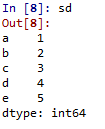Dictionary type data
```

Code #4:When Data contains Ndarray

 `# Program to Create ndarray series`` ` `# Defining 2darray``Data ``=``[[``2``, ``3``, ``4``], [``5``, ``6``, ``7``]]  `` ` `# Creating series of 2darray``snd ``=` `pd.Series(Data)    `

Output:

```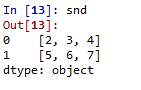Data as Ndarray
```

### DataFrames:

DataFrames is two-dimensional(2-D) data structure defined in pandas which consists of rows and columns.

Code #1: Creation of DataFrame

 `# Program to Create DataFrame`` ` `# Import Library``import` `pandas as pd   `` ` `# Create DataFrame with Data``a ``=` `pd.DataFrame(Data)  `

Here, Data can be:

1. One or more dictionaries
2. One or more Series
3. 2D-numpy Ndarray

Code #2: When Data is Dictionaries

 `# Program to Create Data Frame with two dictionaries`` ` `# Define Dictionary 1``dict1 ``=``{``'a'``:``1``, ``'b'``:``2``, ``'c'``:``3``, ``'d'``:``4``}   `` ` `# Define Dictionary 2     ``dict2 ``=``{``'a'``:``5``, ``'b'``:``6``, ``'c'``:``7``, ``'d'``:``8``, ``'e'``:``9``} `` ` `# Define Data with dict1 and dict2``Data ``=` `{``'first'``:dict1, ``'second'``:dict2} `` ` `# Create DataFrame ``df ``=` `pd.DataFrame(Data)  `

Output:

```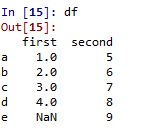DataFrame with two dictionaries
```

Code #3: When Data is Series

 `# Program to create Dataframe of three series ``import` `pandas as pd`` ` `# Define series 1``s1 ``=` `pd.Series([``1``, ``3``, ``4``, ``5``, ``6``, ``2``, ``9``])   `` ` `# Define series 2       ``s2 ``=` `pd.Series([``1.1``, ``3.5``, ``4.7``, ``5.8``, ``2.9``, ``9.3``]) `` ` `# Define series 3``s3 ``=` `pd.Series([``'a'``, ``'b'``, ``'c'``, ``'d'``, ``'e'``])     `` ` `# Define Data``Data ``=``{``'first'``:s1, ``'second'``:s2, ``'third'``:s3} `` ` `# Create DataFrame``dfseries ``=` `pd.DataFrame(Data)              `

Output:

```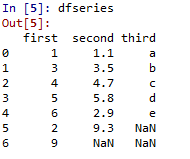DataFrame with three series
```

Code #4: When Data is 2D-numpy ndarray
Note: One constraint has to be maintained while creating DataFrame of 2D arrays – Dimensions of 2D array must be same.

 `# Program to create DataFrame from 2D array`` ` `# Import Library``import` `pandas as pd `` ` `# Define 2d array 1``d1 ``=``[[``2``, ``3``, ``4``], [``5``, ``6``, ``7``]] `` ` `# Define 2d array 2``d2 ``=``[[``2``, ``4``, ``8``], [``1``, ``3``, ``9``]] `` ` `# Define Data``Data ``=``{``'first'``: d1, ``'second'``: d2}  `` ` `# Create DataFrame``df2d ``=` `pd.DataFrame(Data)    `

Output:

```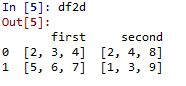DataFrame with 2d ndarray
```

My Personal Notes arrow_drop_up